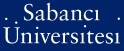# An integral equation based numerical solution for nanoparticles illuminated with collimated and focused light

Şendur, Kürşat (2009) An integral equation based numerical solution for nanoparticles illuminated with collimated and focused light. Optics express, 17 (9). pp. 7419-7430. ISSN 1094-4087Preview
PDF (Open Access) - Requires a PDF viewer such as GSview, Xpdf or Adobe Acrobat Reader
460Kb

Official URL: http://dx.doi.org/10.1364/OE.17.007419

## Abstract

To address the large number of parameters involved in nanooptical problems, a more efficient computational method is necessary. An integral equation based numerical solution is developed when the particles are illuminated with collimated and focused incident beams. The solution procedure uses the method of weighted residuals, in which the integral equation is reduced to a matrix equation and then solved for the unknown electric field distribution. In the solution procedure, the effects of the surrounding medium and boundaries are taken into account using a Green’s function formulation. Therefore, there is no additional error due to artificial boundary conditions unlike differential equation based techniques, such as finite difference time domain and finite element method. In this formulation, only the scattering nano-particle is discretized. Such an approach results in a lesser number of unknowns in the resulting matrix equation. The results are compared to the analytical Mie series solution for spherical particles, as well as to the finite element method for rectangular metallic particles. The Richards-Wolf vector field equations are combined with the integral equation based formulation to model the interaction of nanoparticles with linearly and radially polarized incident focused beams.

Item Type: Article UNSPECIFIED 11588 Kürşat Şendur 20 Jul 2009 16:43 01 Apr 2020 16:56

Repository Staff Only: item control page